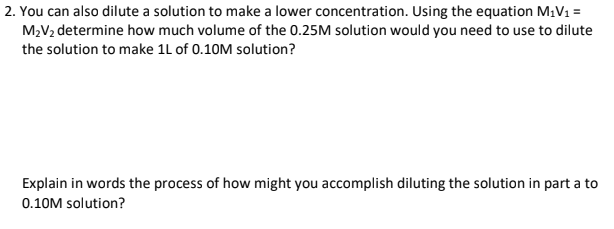# 2. You can also dilute a solution to make a lower concentration. Using the equation M1V1 = M2V2 determine how much volume of the 0.25M solution would you need to use to dilute the solution to make 1L of 0.10M solution? Explain in words the process of how might you accomplish diluting the solution in part a to 0.10M solution?